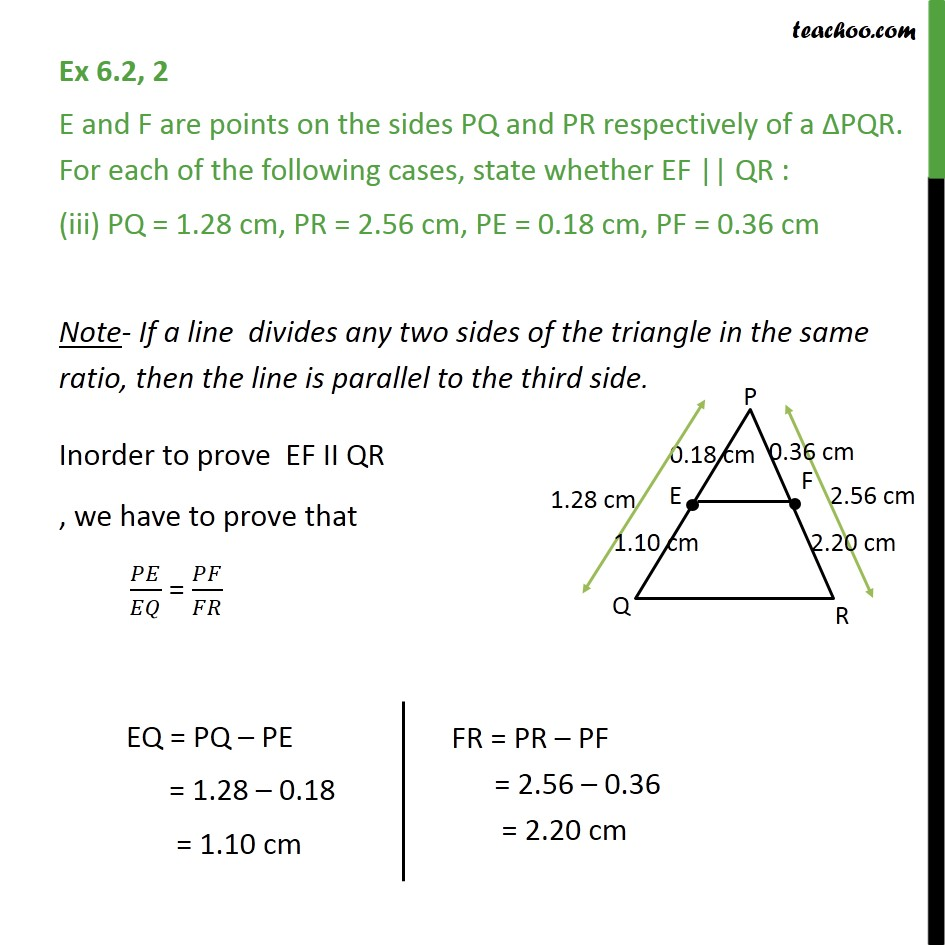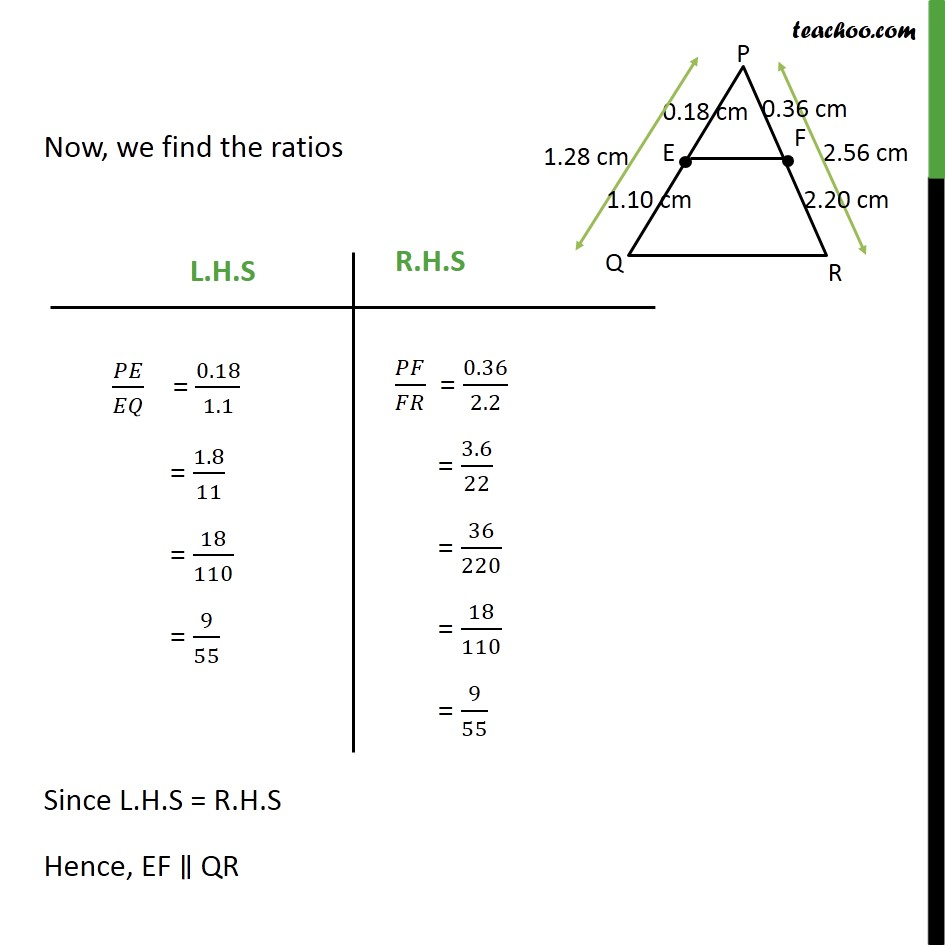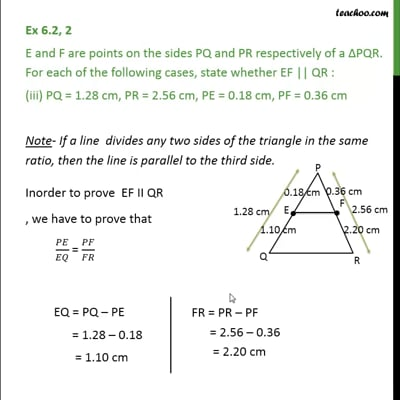Ex 6.2

Chapter 6 Class 10 Triangles
Serial order wiseThis video is only available for Teachoo black users

Learn in your speed, with individual attention - Teachoo Maths 1-on-1 Class

### Transcript

Ex 6.2, 2 E and F are points on the sides PQ and PR respectively of a PQR. For each of the following cases, state whether EF || QR : (iii) PQ = 1.28 cm, PR = 2.56 cm, PE = 0.18 cm, PF = 0.36 cm Note- If a line divides any two sides of the triangle in the same ratio, then the line is parallel to the third side. Inorder to prove EF II QR , we have to prove that / = / Now, we find the ratios Since L.H.S = R.H.S Hence, EF QR